### Home > CALC > Chapter 7 > Lesson 7.2.2 > Problem7-78

7-78.
1.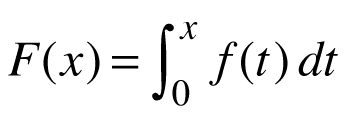for the function f(t) graphed at right. Homework Help ✎

1. When is F(x) at a maximum on [0, 15]?

2. Is F(x) increasing or decreasing or both on [0, 8]?

3. Find F(4), F(10), and F(15).

4. List the interval(s) on [0, 15] for which F ″(x) > 0.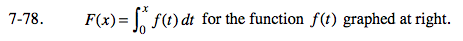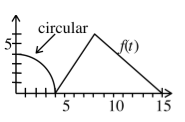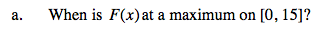An integral represents the area under a curve. At which point is there the largest area?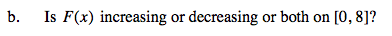Strictly Increasing. (Justify.)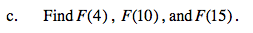$F(10)=\int_{0}^{10}f(t)dt=\text{quarter circle + triangle + trapezoid}$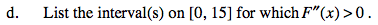Since F '(x) = f(x),
F ''(x) = f '(x).